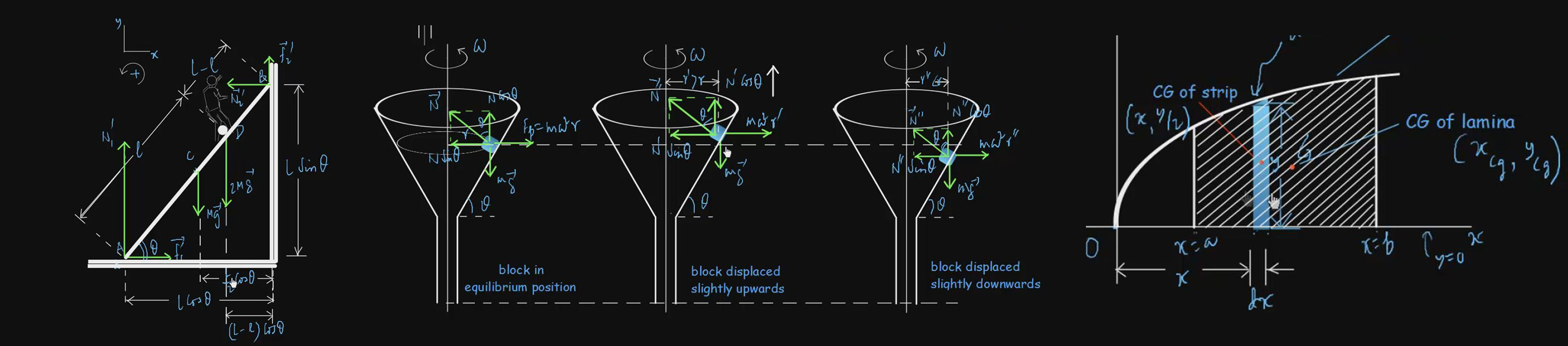# Vectors

A quantity which is completely specified by a number with an appropriate unit is called a scalar. A scalar has only magnitude but no direction. Some scalars like volume and mass are always positive. Other scalars like temperature and electric charge can be either positive or negative. A quantity which is completely specified by a number with an appropriate unit and direction is called a vector. Thus, a vector has both magnitude and direction. Examples of vectors are velocity, acceleration, force, momentum, torque etc. We have video lectures on Addition of Vectors, Subtraction of Vectors, Resolution of Vectors, Product of Vectors and other aspects of vector with suitable examples and problems.

#### Basic level videos

300 5
Addition Of Vectors By Resolution 1:06:46 Basic
350 7.5
Examples Of Vector Addition And Subtraction 49:20 Basic
300 5
Problems On Scalar Product Of Vectors 35:34 Basic
200 3
Problems On Scalar Product Of Vectors II 36:21 Basic
200 3
Problems On Vector Product Of Vectors 43:01 Basic
250 4
Resolution of a position vector in space 1:20:27 Basic
350 7.5
Resolution Of Vector 57:58 Basic
300 5
Scalar Product Of Two Vectors 1:22:27 Basic
350 7.5
Some Important Definitions On Vector 1:00:04 Basic
300 5
Subtraction Of Vectors 38:49 Basic
200 3
Vector Product Of Two Vectors 1:33:10 Basic
400 8
Concept Of Vector 1:02:58 Basic
350 7.5

#### Advanced level Videos Note: (CE) Stands for Problems from Competitive Examination Papers

Advanced-Level Problems On Vectors I 1:06:21
350
7.5